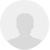# 图解基于FPGA的贪食蛇驱动程序

0
4703 42

今天由我来给大家介绍一下用FPGA来驱动贪食蛇游戏的具体实现方法。

需要的硬件资源有：带4位按键和VGA接口的FPGA最小系统板，还有显示器一台。

分辨率640*480

集成开发环境：quartusII。

HDL：Verilog

U1:  pll  用于将输入的20M晶振倍频为50M供其他模块使用

U2：Game_Ctrl_Unit  控制游戏的四种状态,之间的转换

U3: Snake_Eatting_Apple  随机产生苹果

U4：Snake  产生各个VGA扫描部件的坐标及控制蛇运动轨迹

U5：VGA_Control VGA扫描控制模块

U6：Key 键盘扫描模块

U7：Seg_Display 数码管显示计分模块U1：PllU6：Keyup_key_press<=0;

up_key_last<=0;

U2：Game_Ctrl_UnitU4:Snakeinput left_press,

input right_press,

input up_press,

input down_press,//按键输入

output reg [1:0]snake,//用于表示当前扫描扫描的部件四种状态00：NONE 01：HEAD 10：BODY 11：WALL

input [9:0]x_pos,

input [9:0]y_pos,//扫描坐标  单位：“像素点”

input [1:0]game_status,//四种游戏状态

output reg [6:0]cube_num,

output reg hit_body,

output reg hit_wall,

input die_flash

......

reg [5:0]cube_x[15:0];
reg [5:0]cube_y[15:0];//体长坐标 单位：“格子”if(x_pos[9:4]==0|y_pos[9:4]==0|x_pos[9:4]==39|y_pos[9:4]==29)

snake=WALL;//扫描墙reg [5:0]cube_x[15:0];
reg [5:0]cube_y[15:0];

is_exist有16位，即蛇体最长为16*1格，每一位对应一个格，1为该格显示，0则不显示（图中虚框）

always@(posedge CLK_50M or negedge RSTn)

if(cnt==12_500_000) //0.02us*12'500'000=0.25s   每秒移动四次

….

if(game_status==PLAY)

……

begin

cube_x<=cube_x;

cube_y<=cube_y;

cube_x<=cube_x;

cube_y<=cube_y;

cube_x<=cube_x;

cube_y<=cube_y;

cube_x<=cube_x;

cube_y<=cube_y;

….

…

end

if((cube_y==cube_y&&cube_x==cube_x&&is_exist==1)|

(cube_y==cube_y&&cube_x==cube_x&&is_exist==1)|

(cube_y==cube_y&&cube_x==cube_x&&is_exist==1)|

(cube_y==cube_y&&cube_x==cube_x&&is_exist==1)|

……）

hit_body<=1；

//头的格Y坐标=任一位身体的格Y坐标且头的格X坐标=任一位身体的格X坐标且身体的该长度位存在  判定为hit_body

case(direct)

UP:

begin

if(cube_y==1)

hit_wall<=1;

else

cube_y<=cube_y-1;

end

DOWN:

……

LEFT:

……

RIGHT

……

endcase

……

0:begin

begin

cube_num<=cube_num+1;

is_exist[cube_num]<=1;

end

end

1:begin

end

if(x_pos>=0&&x_pos<640&&y_pos>=0&&y_pos<480)

begin

if(x_pos[9:4]==0|y_pos[9:4]==0|x_pos[9:4]==39|y_pos[9:4]==29)

snake=WALL;//扫描wall

else if(x_pos[9:4]==cube_x&&y_pos[9:4]==cube_y&&is_exist==1)

begin

end

else if

((x_pos[9:4]==cube_x&&y_pos[9:4]==cube_y&&is_exist==1)|

(x_pos[9:4]==cube_x&&y_pos[9:4]==cube_y&&is_exist==1)|

(x_pos[9:4]==cube_x&&y_pos[9:4]==cube_y&&is_exist==1)|

(x_pos[9:4]==cube_x&&y_pos[9:4]==cube_y&&is_exist==1)|

(x_pos[9:4]==cube_x&&y_pos[9:4]==cube_y&&is_exist==1)|

(x_pos[9:4]==cube_x&&y_pos[9:4]==cube_y&&is_exist==1)|

……（身体有十六位..）

snake=(die_flash==1)?BODY:NONE;//扫描body

else snake=NONE;

end

end

U3：Snake_Eatting_Applealways@(posedge CLK_50M)

random_num<=random_num+927;  //用加法产生随机数

//随机数高5位为apple格X坐标低5位为apple格Y坐标

if(clk_cnt==250_000)

……

begin

apple_x<=(random_num[10:5]>38)?(random_num[10:5]-25):(random_num[10:5]==0)?1:random_num[10:5];

apple_y<=(random_num[4:0]>28)?(random_num[4:0]-3):(random_num[4:0]==0)?1:random_num[4:0];

end   //判断随机数是否超出屏幕坐标范围将随机数转换为下个apple的X Y坐标

U5：VGA_Controlalways@(posedge CLK_50M)

begin

clk_25M<=~clk_25M;  //2分频   按25M配置VGA控制模块

end

VGA的hsync和vsync控制时序如下，XD，具体的VGA驱动原理我就不多讲咯~不了解VGA驱动原理的同学做这个游戏之前最好先了解一下。当然，仅凭上面几张图还有源代码就看懂的同学就太牛B了~~

U7：Seg_Display1.商周 42#

有时间学习一下。mark...请问大家下下来可以运行吗？为什么我这里显示器不亮？

2.DSX 41#

请问大家下下来可以运行吗？为什么我这里显示器不亮？

3.xzy610030 40#
有时间学习一下。mark...
4.yissen 39#
嗯 给力 学习了！
5.1010226353 38#
7 楼okokxinxinxi  能说一下你的输入输出接口都代表什么吗？
6.jeremy2013 37#

学习学习学习！

7.wswxdw 36#
想看看代码
8.fcten 35#

路过学习9.liyinbc779 34#

这正是我需要的

10.Cosmo 33#

必须得试一下！

11.veryshi 32#

参考参考

12.jewelyang 31#

thx!

13.lizihe123 30#

试试~！

14.canwu 29#

果断的学习学习

15.zhoubin7020 28#

下来学习一下